SubSection CCD.MVT The Mean Value Theorem

Theorem CCD.MVT. The Mean Value Theorem:
If $f$ is a continuous function on the interval $[a,b]$ and a differentiable function on the interval $(a,b)$, then there is a number $c \in (a,b)$  where  $$f'(c) = \frac{f(b) - f(a)}{b-a}$$ or $$f(b) = f(a) + f'(c) (b-a)$$ .

The Mean Value Theorem and 1st Derivative Analysis:
The analysis of the first derivative, traditionally treated using graphs, is visualized in this section with mapping diagrams. Extremes and critical numbers and values are connected to mapping diagrams.
CCD.MVT.FDA : First Derivative Analysis. Visualizing the derivative for an interval with the "derivative vector" in a mapping diagram supports first derivative analysis for monotonic function behavior.
Mapping Diagram Examples: $1:x^2; 2: 3x^2-2x^3 ; 3: 2x^3-3x^2; 4: \sin(\frac {\pi}2 x)$
This is a Java Applet created using GeoGebra from www.geogebra.org - it looks like you don't have Java installed, please go to www.java.com
Graphs of functions and mapping diagrams visualize first derivative analysis.

The Mean Value Theorem and 2nd Derivative Analysis:
CCD.MVT.SDA : Second Derivative Analysis. The analysis of the second derivative, traditionally treated using graphs, is visualized in this section with mapping diagrams. Using acceleration to interpret the second derivative connects the second derivative analysis to the (rate of) change of the derivative.
If $f''(x) \gt 0$  for an interval then $f'(x)$ is increasing for that interval and $f(x)$ is accelerating for that interval.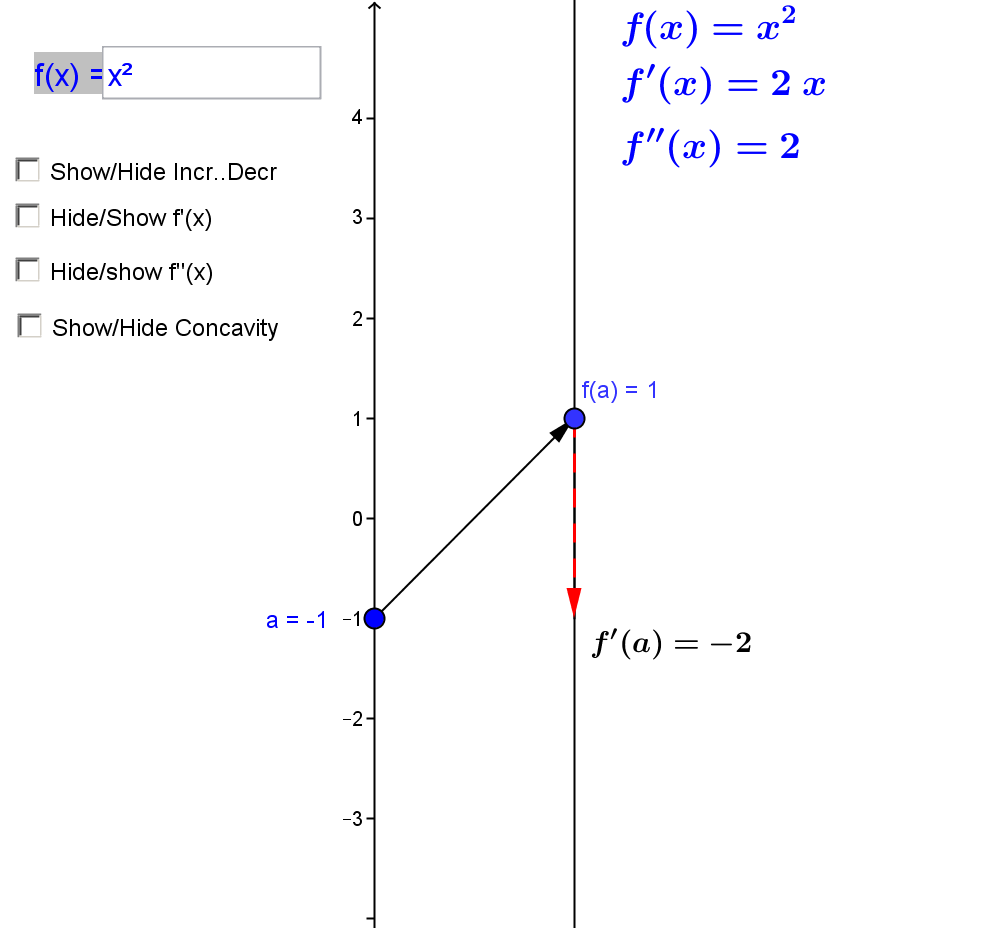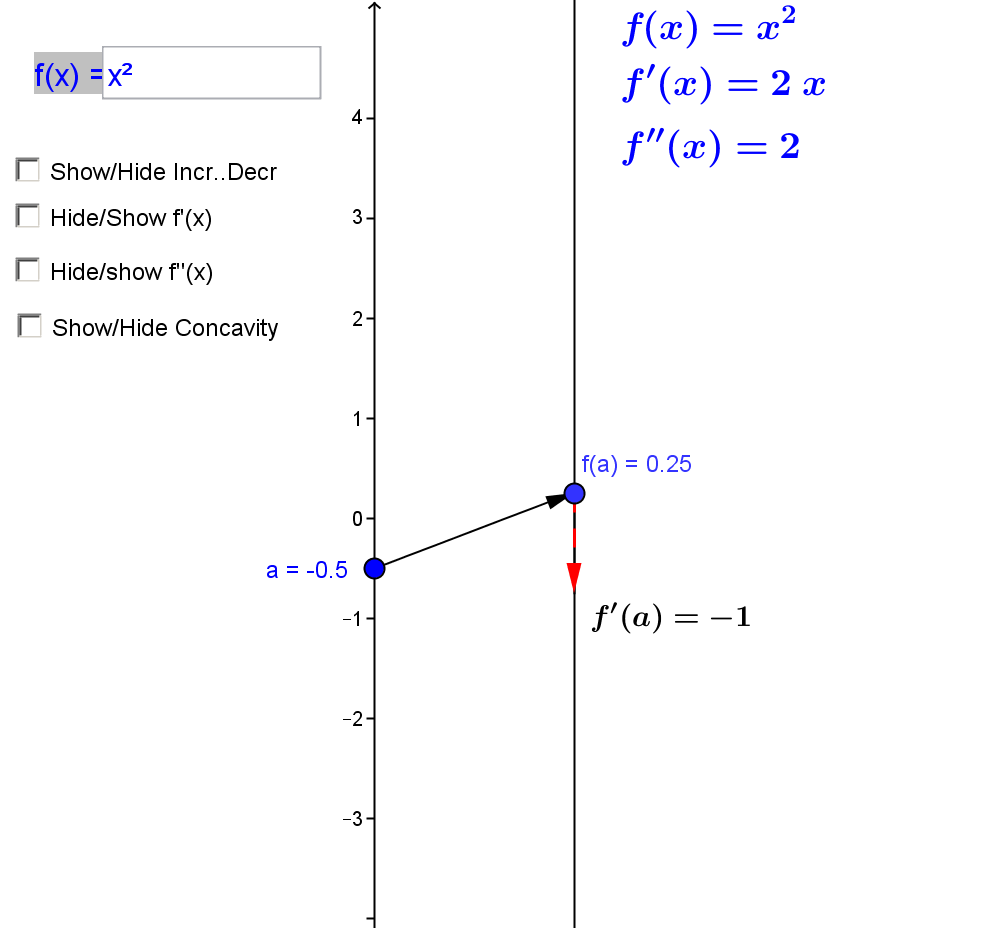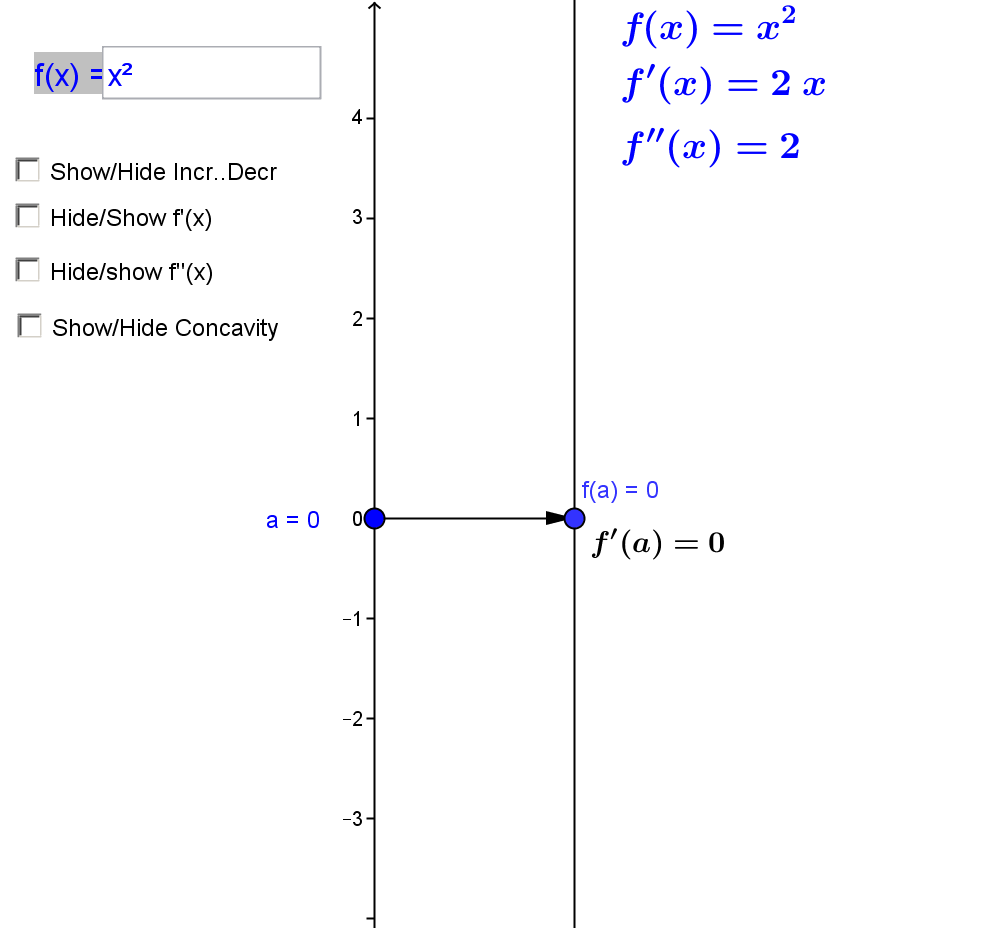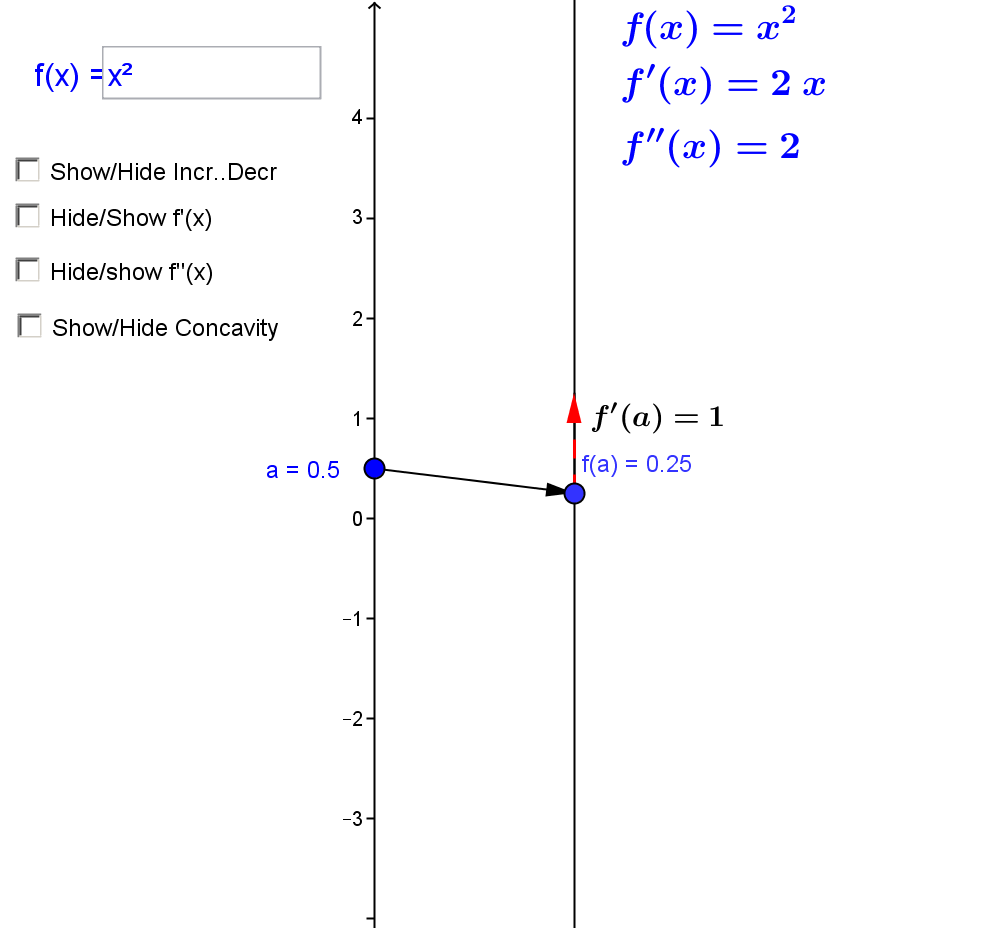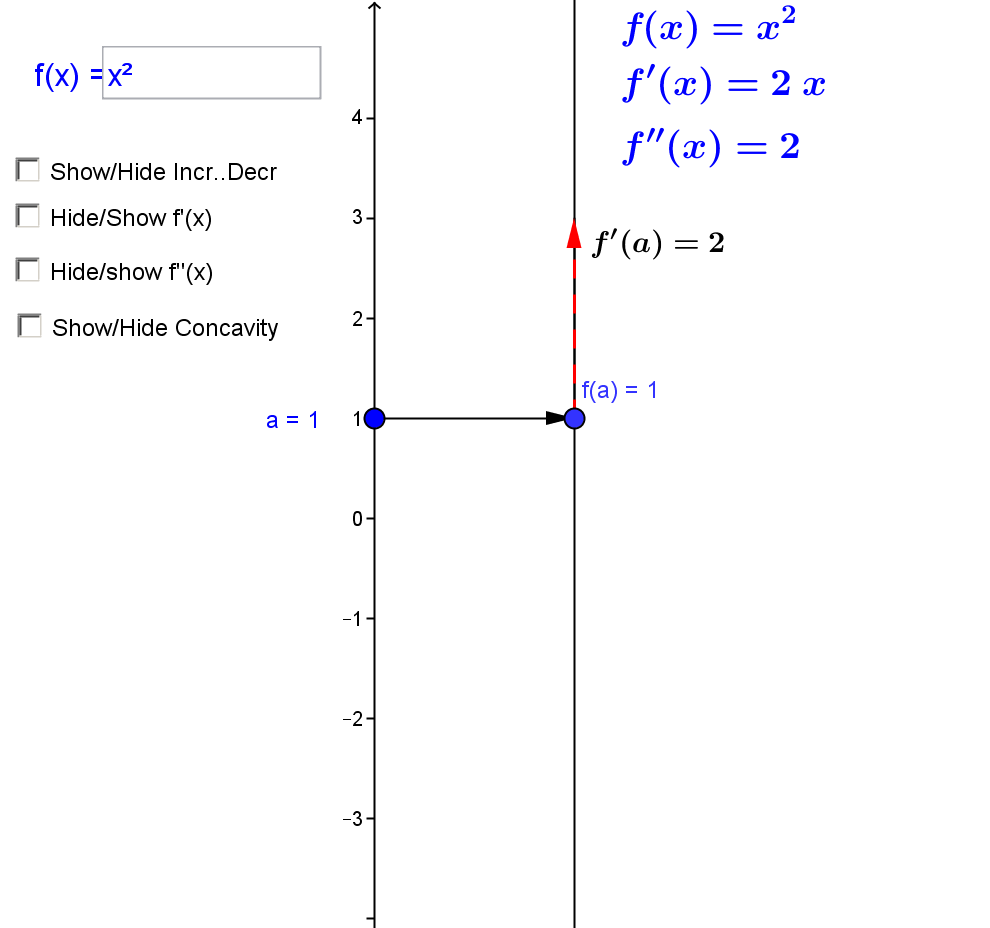This is a Java Applet created using GeoGebra from www.geogebra.org - it looks like you don't have Java installed, please go to www.java.com
Notice how the points on the graph are paired with the arrows on the mapping diagram.

CCD.MVT.SFDA : First and Second Derivative Analysis. Visualizing the derivative for an interval with the "derivative vector" in a mapping diagram supports first derivative analysis for extremes, critical numbers and values, and the first and second derivative tests for extremes.
Mapping Diagram and Graphs for First and Second Derivative Analysis Examples
This is a Java Applet created using GeoGebra from www.geogebra.org - it looks like you don't have Java installed, please go to www.java.com